# A slender 9 lb rod can rotate in a vertical plane about a pivot at B. A spring of constant k-30 lb/ft and of unstretched length 6 in. is attached to the rod as shown. The rod is released from rest in...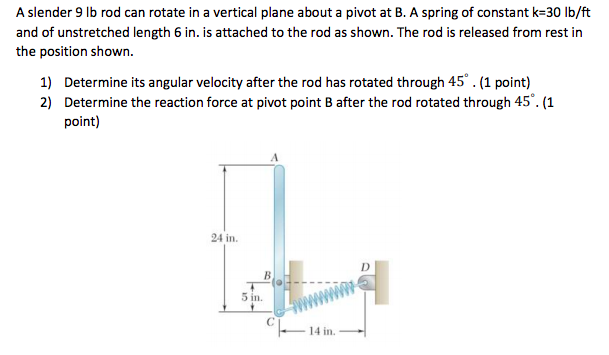A slender 9 lb rod can rotate in a vertical plane about a pivot at B. A spring of constant k-30 lb/ft and of unstretched length 6 in. is attached to the rod as shown. The rod is released from rest in the position shown. 1) Determine its angular velocity after the rod has rotated through 45.(1 point) 2) Determine the reaction force at pivot point B after the rod rotated through 45. (1 point) 24 in 5 in. 4 in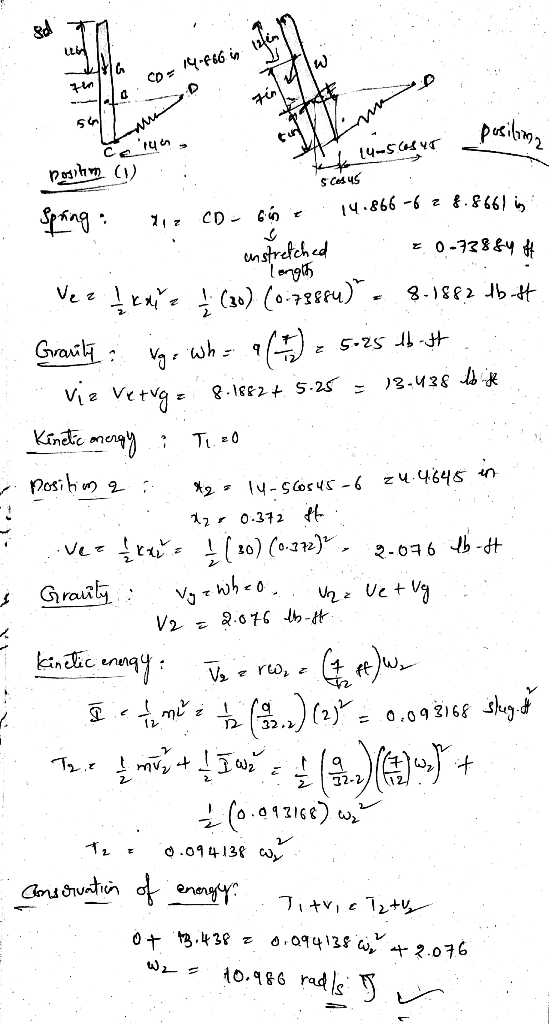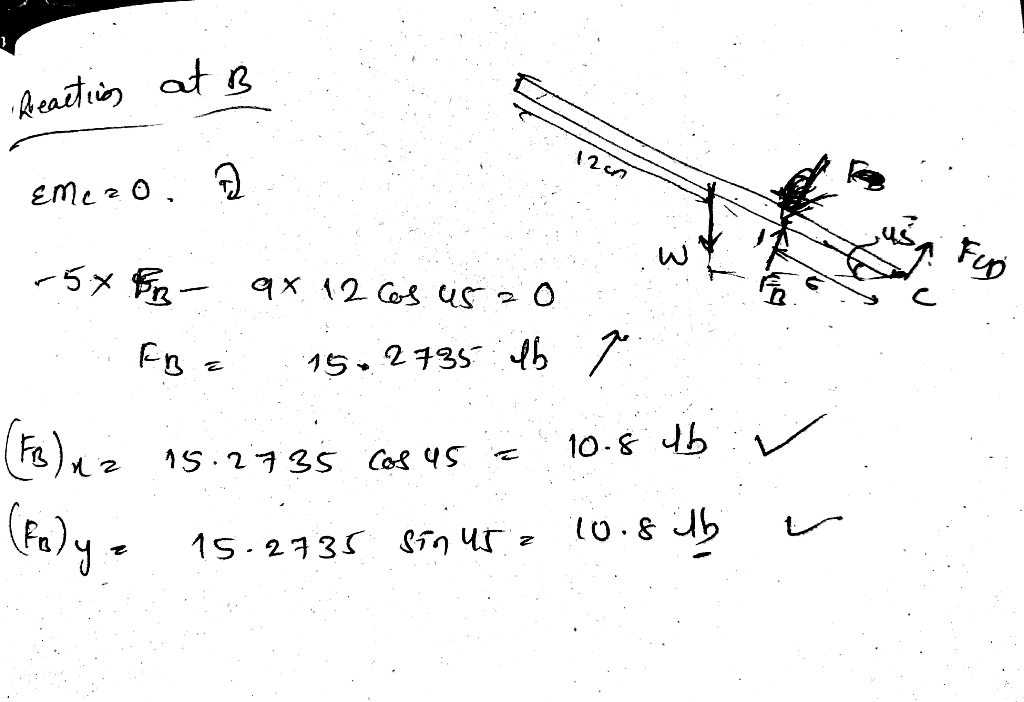##### Add Answer of: A slender 9 lb rod can rotate in a vertical plane about a pivot at B. A spring of constant k-30 lb/ft and of unstretched length 6 in. is attached to the rod as shown. The rod is released from rest in...
Similar Homework Help Questions
• ### Problem 9 (15 points) A 20-lb slender rod AB is 5 ft long and is pivoted about a point O which is...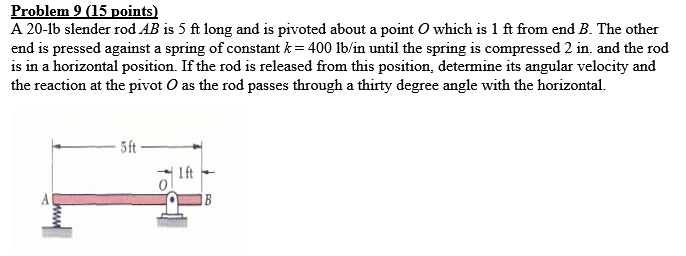Problem 9 (15 points) A 20-lb slender rod AB is 5 ft long and is pivoted about a point O which is 1 ft from end B. The other end is pressed against a spring of constant k-400 lbin until the spring is compressed 2 in, and the rod is in a horizontal position. If the rod is released from this position, determine its angular velocity and the reaction at the pivot O as the rod passes through a thirty...

• ### A 24 lb uniform slender rod AB of length L= 36 in is fixed to a...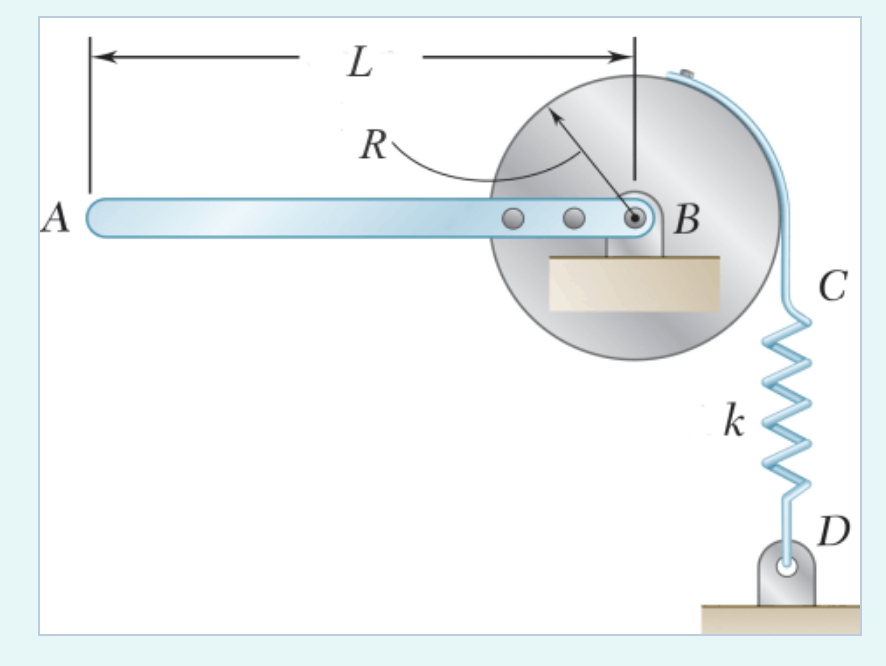A 24 lb uniform slender rod AB of length L= 36 in is fixed to a 12 lb uniform disk of radius, R= 12 in , and both are pinned at B as shown. A belt is attached to the rim of the disk and then to a spring with spring constant, k= 40 lb/in which holds the rod/disk at rest in the equilibrium position shown. natural period is 0.446 s If end A is displaced a distance of 0.9...

• ### Sample Problem 6. The 30 kg slender rod is released from rest when θ-00 (when rod OA is in the horizontal position). The spring is unstretched at θ-00. A constant clockwise moment of 10 N-m is applie...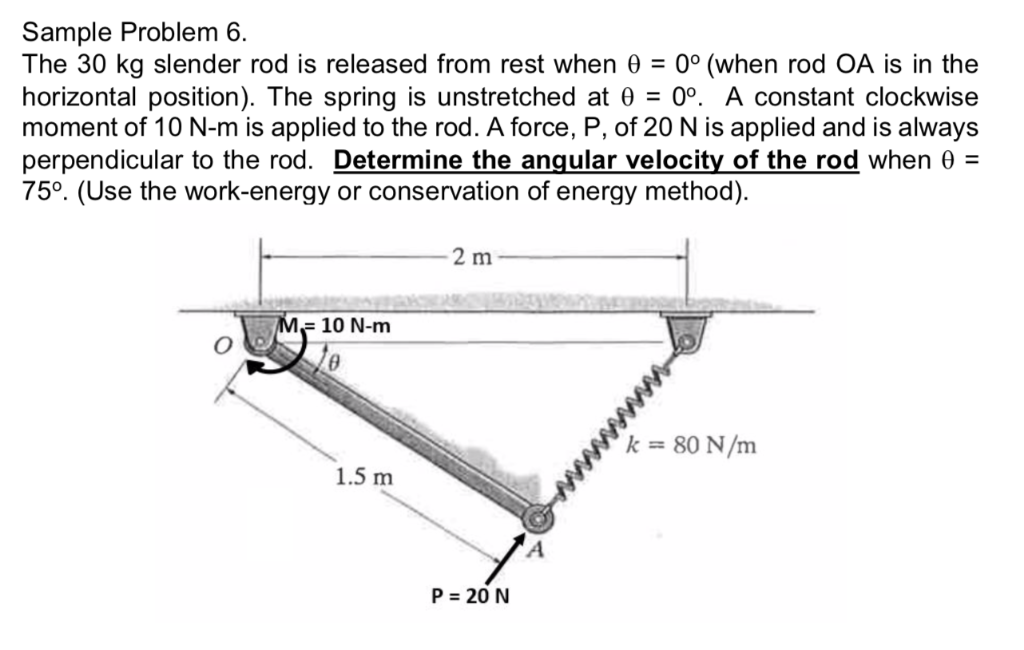Sample Problem 6. The 30 kg slender rod is released from rest when θ-00 (when rod OA is in the horizontal position). The spring is unstretched at θ-00. A constant clockwise moment of 10 N-m is applied to the rod. A force, P, of 20 N is applied and is always perpendicular to the rod. Determine the angular velocity of the rod when θ- 750. (Use the work-energy or conservation of energy method). 2 m 10 N-mm k80 N/m 1.5...

• ### 4. The pendulum in Figure 4 consists of a 10 lb sphere of radius 1.0 f and a 5 lb slender rod of length 2.0 ft. Determine: (10 pts) the angularacceleration of the pendulum immediately after th...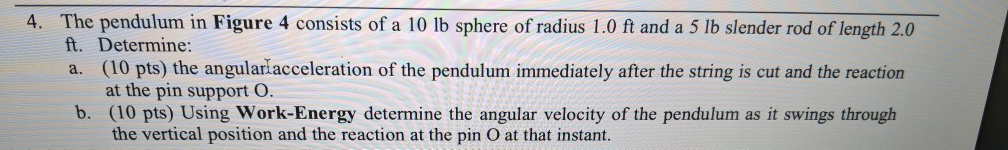4. The pendulum in Figure 4 consists of a 10 lb sphere of radius 1.0 f and a 5 lb slender rod of length 2.0 ft. Determine: (10 pts) the angularacceleration of the pendulum immediately after the string is cut and the reaction at the pin support O a. b. (10 pts) Using Work-Energy determine the angular velocity of the pendulum as it swings through the vertical position and the reaction at the pin O at that instant. BC 1...

• ### B Classwork for Dynamics Online x M Inbox (369)- [email protected] xChegg Study | Guided Solutions x...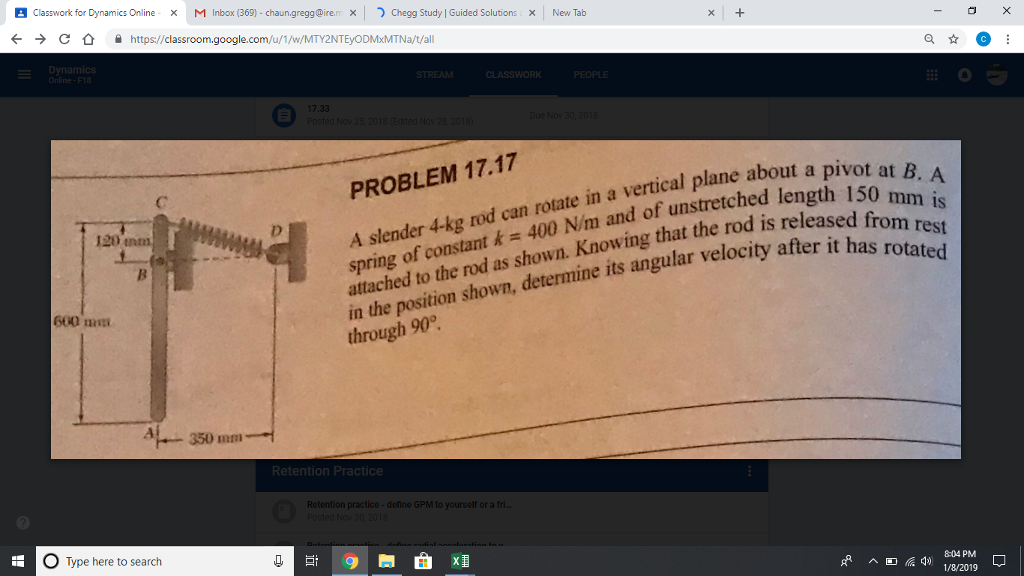B Classwork for Dynamics Online x M Inbox (369)- [email protected] xChegg Study | Guided Solutions x New Taib < + Chttps://classroom.google.com/u/1/w/MTY2NTEyODMxMTNa/t/all A slender 4-kg rod can rotate in a vertical plane about spring of constant k400 N/m and of unstretched len attached to the rod as shown. Knowing that the rod is in the position shown, determine its angular veloci through 90° PROBLEM 17.17 a pivot at B. A 150 mm is 120 nm atter it has rotated 600 mmp...

• ### B Classwork for Dynamics Online x M Inbox (369)- [email protected] xChegg Study | Guided Solutions x...B Classwork for Dynamics Online x M Inbox (369)- [email protected] xChegg Study | Guided Solutions x New Taib < + Chttps://classroom.google.com/u/1/w/MTY2NTEyODMxMTNa/t/all A slender 4-kg rod can rotate in a vertical plane about spring of constant k400 N/m and of unstretched len attached to the rod as shown. Knowing that the rod is in the position shown, determine its angular veloci through 90° PROBLEM 17.17 a pivot at B. A 150 mm is 120 nm atter it has rotated 600 mmp...

• ### Need some help with this spring problemA 3-kg slender rod rotates in a vertical plane about a pivot atB.A spring of constant k=300 N/m and of unstretched length120mm is attached to the rod asshown.Knowing that in theposition shown the rod has an angular velocity of 4 rad/sclockwise, determine the angular velocity of the rod after it hasrotatedthrough a) 90o, b) 180oAC=750mm, BC=150mm, BD=360mm

• ### Chapter 17A slender 6-kg rod can rotate in a vertical plane about apivot at B .a spring of constant k=600N/m and an unstreched lengthof 225 mm is attached to the rod asshown. knowing that the rod isreleased from rest in the position shown,determine its angularvelocity after it has rotated through 90o.

• ### A 12 kg slender rod is placed against the wall at an angle of 45 degrees as shown. If it is released from rest, determine the angular velocity of the rod at the instant just before it hits the gr...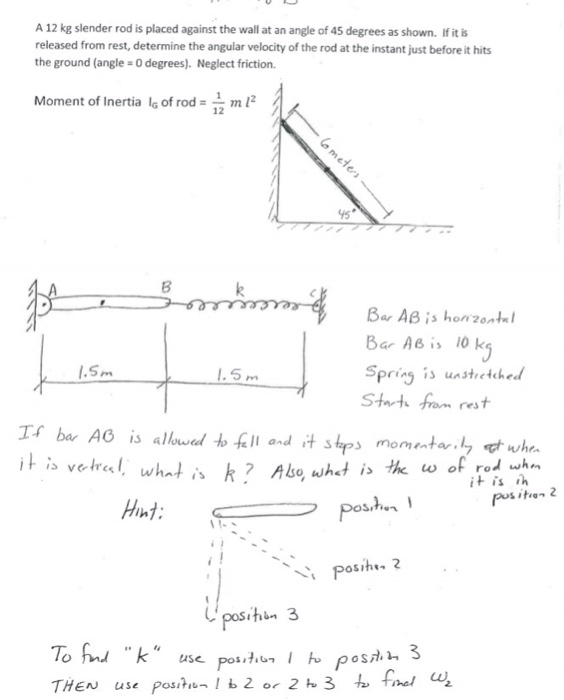A 12 kg slender rod is placed against the wall at an angle of 45 degrees as shown. If it is released from rest, determine the angular velocity of the rod at the instant just before it hits the ground (angle 0 degrees). Neglect friction. Moment of Inertia lg of rod-2 12 8 Bar AB is l0 k Spring unstrctched Starth from rest Hint: Tou k" A 12 kg slender rod is placed against the wall at an angle of...

• ### A thin rod, of length L and negligible mass, that can pivot about one end to rotate in a vertical circle

A thin rod, of length L and negligible mass, that can pivot about one end to rotate in a vertical circle. A heavy ball of mass m is attached to the other end. The rod is pulled aside through an angle and released. (a)What is the speed of the ball at the lowest point if L = 2.20 m, = 19.0°, and m = 500 kg? (b) Does the speed increase, decrease, or remain the same if the mass is...

Need Online Homework Help?